Background

Canonical correlation was developed by Hotelling (1935, 1936).

The application of canonical correlation is discussed by Cooley and Lohnes (1971); Tatsuoka (1971); Mardia, Kent, and Bibby (1979). One of the best theoretical treatments is given by Kshirsagar (1972).

Given a set of pvariables and q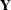variables, the CANCORR procedure finds the linear combinations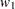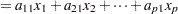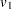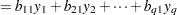such that the two canonical variables,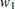and, have the largest possible correlation. This maximized correlation between the two canonical variables is the first canonical correlation. The coefficients of the linear combinations are canonical coefficients or canonical weights. It is customary to normalize the canonical coefficients so that each canonical variable has a variance of 1.

PROC CANCORR continues by finding a second set of canonical variables, uncorrelated with the first pair, that produces the second-highest correlation coefficient. That is, the second pair of canonical variables is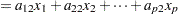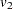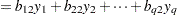such that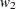is uncorrelated withand,is uncorrelated withand, andandhave the largest possible correlation subject to these constraints. The process of constructing canonical variables continues until the number of pairs of canonical variables is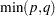, the number of variables in the smaller group.

Each canonical variable is uncorrelated with all the other canonical variables of either set except for the one corresponding canonical variable in the opposite set. The canonical coefficients are not generally orthogonal, however, so the canonical variables do not represent jointly perpendicular directions through the space of the original variables.

The first canonical correlation is at least as large as the multiple correlation between any variable and the opposite set of variables. It is possible for the first canonical correlation to be very large while all the multiple correlations for predicting one of the original variables from the opposite set of canonical variables are small. Canonical redundancy analysis (Stewart and Love; 1968; Cooley and Lohnes; 1971; van den Wollenberg; 1977) examines how well the original variables can be predicted from the canonical variables.

PROC CANCORR can also perform partial canonical correlation, which is a multivariate generalization of ordinary partial correlation (Cooley and Lohnes; 1971; Timm; 1975). Most commonly used parametric statistical methods, ranging from t tests to multivariate analysis of covariance, are special cases of partial canonical correlation.